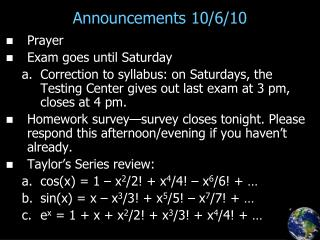DownloadDownload PresentationAnnouncements 10/6/10

# Announcements 10/6/10

Télécharger la présentation## Announcements 10/6/10

- - - - - - - - - - - - - - - - - - - - - - - - - - - E N D - - - - - - - - - - - - - - - - - - - - - - - - - - -
##### Presentation Transcript

1. Announcements 10/6/10 • Prayer • Exam goes until Saturday • Correction to syllabus: on Saturdays, the Testing Center gives out last exam at 3 pm, closes at 4 pm. • Homework survey—survey closes tonight. Please respond this afternoon/evening if you haven’t already. • Taylor’s Series review: • cos(x) = 1 – x2/2! + x4/4! – x6/6! + … • sin(x) = x – x3/3! + x5/5! – x7/7! + … • ex = 1 + x + x2/2! + x3/3! + x4/4! + …

2. Reminder • What is w? • What is k? • How do they relate to the velocity? • Relationship between w and T • Relationship between k and l • Consistency check: w/k = ?

3. Reading Quiz • What’s the complex conjugate of:

4. Complex Numbers – A Summary What is the square root of 1… 1 or -1? • What is “i”? • What is “-i”? • The complex plane • Complex conjugate • Graphically, complex conjugate = ? • Polar vs. rectangular coordinates • Angle notation, “Aq” • Euler’s equation…proof that eiq = cosq + isinq •  must be in radians • Where is 10ei(p/6) located on complex plane?

5. Complex Numbers, cont. • Adding • …on complex plane, graphically? • Multiplying • …on complex plane, graphically? • How many solutions are there to x2=1? • What are the solutions to x5=1? (xxxxx=1) • Subtracting and dividing • …on complex plane, graphically?

6. Polar/rectangular conversion • Warning about rectangular-to-polar conversion: tan-1(-1/2) = ? • Do you mean to find the angle for (2,-1) or (-2,1)? Always draw a picture!!

7. Using complex numbers to add sines/cosines • Fact: when you add two sines or cosines having the same frequency (with possibly different amplitudes and phases), you get a sine wave with the same frequency! (but a still-different amplitude and phase) • “Proof” with Mathematica… (class make up numbers) • Worked problem: how do you find mathematically what the amplitude and phase are? • Another worked problem?

8. Using complex numbers to solve equations • Simple Harmonic Oscillator (ex.: Newton 2nd Law for mass on spring) • Guess a solution like what it means, really: (and take Re{ … } of each side)

9. Complex numbers & traveling waves • Traveling wave: A cos(kx – wt + f) • Write as: • Often: • …or • where “A-tilde” = a complex number, the phase of which represents the phase of the wave • often the tilde is even left off